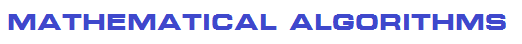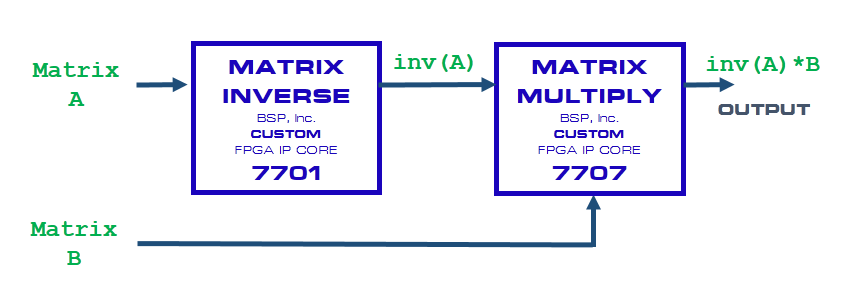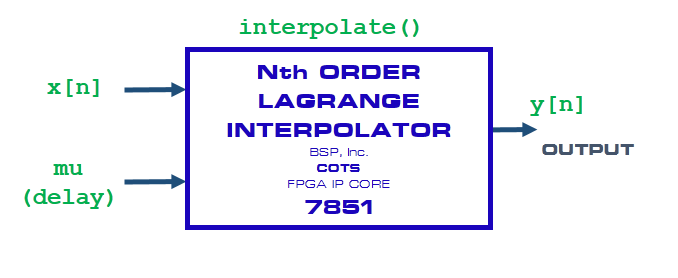Below is an example combining Blueprint Signal Processing FPGA IP Cores to implement matrix mathematics:Mathematical Algorithms form the basis of signal processing technology.

Blueprint Signal Processing FPGA IP Cores implement a wide range of fixed point or floating point mathematical functions to support high performance, end-to-end signal processing:

• Matrix and Vector Mathematical Functions (Matrix Inversion, etc.)
• Trigonometric Functions
• Statistical Math Functions
• Calculus and Geometric Functions
• Differential Equations
• Special Functions

Blueprint Signal Processing FPGA IP Cores can implement a wide range of mathematical and signal processing functions, below is Lagrange Interpolator FPGA IP Core: